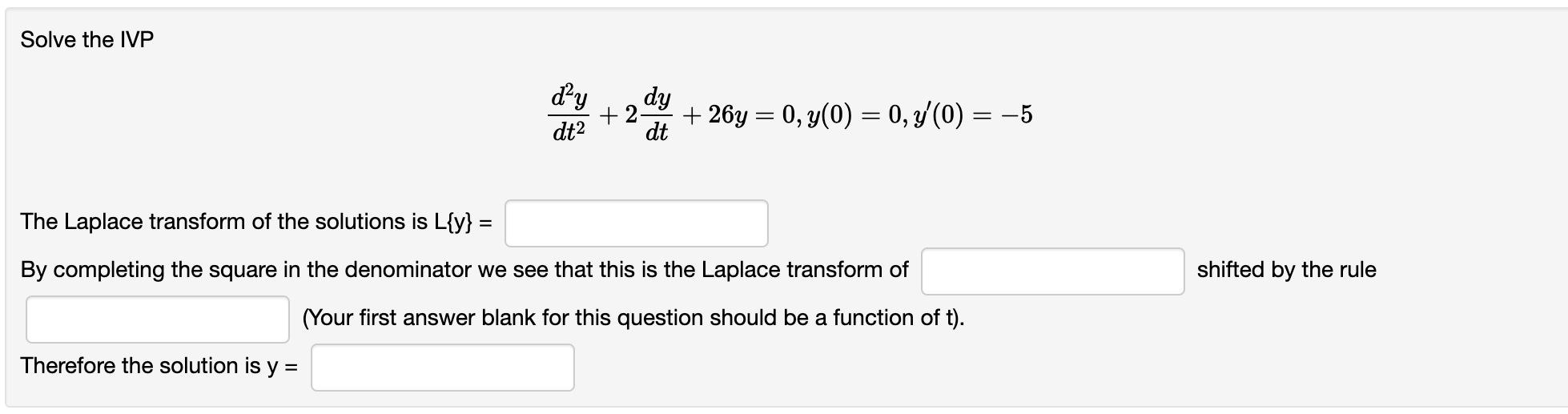# Question Solve the IVP dy dy +2 +26y = 0, y(0) = 0, y(0) = -5 dt2 dt = = The Laplace transform of the solutions is L{y} = = By completing the square in the denominator we see that this is the Laplace transform of shifted by the rule (Your first answer blank for this question should be a function of t). Therefore the solution is y =